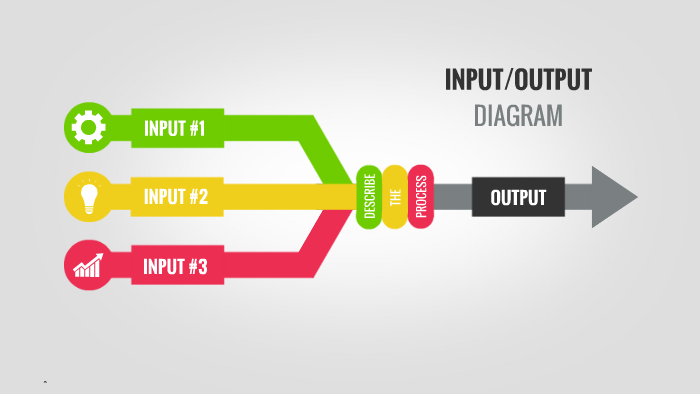# Kuby Block

Blog about programming, tech, society and more.In javascript, there are some situation we only want to call a function with one, two or three parameter(s), but input of it maybe there are a lot of parameters. For example, we want to repeat every element in array one time:

``````
const data = [2, 11, 6, 10];
// expected [22, 1111, 66, 1010]

``````

What will you do?

Simplest, we can do:

``````
const data = [2, 11, 6, 10];

data.map(el => el.toString()).map(el => el.repeat(2)).map(el => Number(el));

// output [22, 1111, 66, 1010]

``````

Yeah, that ok, but we can do something to make it more elegant :)

we have a repeat function like this:

``````
function repeat(inp, times){
if(undefined === times) {
times = 2;
}

let result = "";
for (let i = 0; i < times; ++i) {
result += inp;
}
return result;
}

``````

And …

``````const data = [2, 11, 6, 10];

data.map(Number.prototype.toString).map(repeat).map(Number);

// But ...
// Uncaught TypeError: Function.prototype.apply was called on undefined, which is a undefined and not a function

``````

What happen?

Because `toString` function of class Number can receive more than 1 parameter, so when you call that, it will use second value of map function as radix number.

`map` will put to callback function 3 parameter: value, index and this array

# Resolve it…

We can use a technique to help the function will receive only one parameter, Write `unique` function:

``````
function unique (fn) {
return (...params) => fn.hasOwnProperty("arguments") ? fn(params) : fn.apply(params);
}

``````

`unique` function will help `fn` function invoke with only one parameter,

Now re-write it:

``````
const data = [2, 11, 6, 10];

data.map(unique(Number.prototype.toString)).map(repeat).map(Number);

// (4) [0, 11, 66, 101010]

``````

Soah, what happen?

Problem occurs with `repeat` function as well. So, rewrite it

``````
const data = [2, 11, 6, 10];

data.map(unique(Number.prototype.toString)).map(unique(repeat)).map(Number);
// output (4) [22, 1111, 66, 1010]

``````

Ok, maybe you not feel elegant, but you can consider it as alternative solution to resolve problem if possible :)